# Calculating the Chord Lengths of an n-frequency Icosahedral Geodesic Dome

David Anderson
originally written in January 1998

After getting a copy of BuckyWorks for Christmas, I decided to build a Geodesic Dome with the kids in the basement. A 1-frequency icosahedral dome would be simple, but not very much fun. I wanted more of a construction challenge. A 2- or 3-frequency dome would be cool; fun to build and potentially elaborate. So off to the web I went...

After looking around for some information for a while, I couldn't find any staightforward analysis of the icosahedron. Lots of pictures and drawings, but nothing on chord lengths and connectors so I'd know what I'd have make. Damn.

So I got out my paper, pencil and calculator.

An icosahedron has 12 vertices, 20 faces, and 30 edges: the most elaborate of the five regular polyhedrons. The icosahedron is a good approximation of a sphere: each vertex lies on the surface of the sphere it circumscribes. Structurally, the icosahedron is pure simplicity: each face is an equilateral triangle and all of the edges are the same length. When a higher frequency icosahedral geodesic structure is desired, the structure becomes more complicated; each icosahedral face is tesselated into n2 subfaces. Although the tesselation of the icosahedral face is rotationally symmetric (with a period of 2/3), the sizes of the projected subfaces are not the same; their edges increase in length as the center of the face is approached.

The simplest representation for vertices of the icosahedron (in normal geometric terms) is in polar coordinates. The following table sumarizes the coordinates for an edge length of 1.

 polarcoordinates radius phi(degrees) theta(degrees) top 0.95105 0.0 upperpentagonallayer 63.4356 0 72 144 216 288 lowerpentagonallayer 116.5644 36 108 180 252 324 bottom 180

Where do these values come from? The following analysis is algebraic, with a little triginometry thrown in. If it makes your head spin, you can take take a step back and trust the results.

To keep things clean, I'm going to work in radians from now on. A radian is defined in terms of the ratio of a circle's circumference C and radius r: when a circle has a radius of 1, the circumference is 2. That is, the angular distance around a unit circle is 2radians. This corresponds to the more familiar 360 degrees, leading to the equivalence 2radians = 360 degrees. In the following discussion you can use this equivalence to convert back and forth to degrees if you'd like, but the sooner you embrace radians the easier time you'll have.

The goal of this analysis is to determine the radius, theta, phi for each vertex of the icosahedron.

Each vertex is equidistant from the center of the circumscribed sphere, implying each will have the same value for its radius - it's the radius of the circumscribing sphere, or the circumradius. Consider that the vertices of an icosahedron are paired: each is diametrically opposed to another. If one pair is set to be at the icosahedron's "top" and "bottom", then the other vertices are arranged on two pentagons between them, each pentagon of phase with the other. The theta's simply walk around the top-bottom axis in/5 radian steps. The center of the layers lies on the top-bottom axis. The phis of the top and bottom vertices are 0 andradians, while the phi of the pentagonal vertices alternate between the angle above and below the perpendicular bisecting plane of the top-bottom axis (that's the plane where phi =radians).

The two unknowns of the icosahedron are therefore the radius of the circumscribed sphere (we'll call it d), and the angle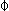(that's phi) which a vertex in a pentagonal layer makes with the top-bottom axis at it's midpoint (that's the center of the of the icosahedron.) The plan is to find the radius d as the distance from the center of the icosahedron to a vertex on a pentagonal layer. Then, by knowing the upper pentagonal layer altitude and the perpendicular distance of a vertex in the upper pentagonal layer from the top-bottom axis, phi may be found for a pentagonal layer vertex.

So mathematically, our target is the blue highlighted figure embedded in the icosahedron below. Again, don't panic; all of the needed computations may be done via simple trigonometry and analytic geometry. We'll even continue to use color-coded diagrams to make things easier to see.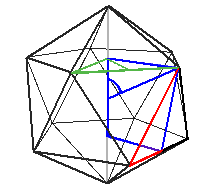First we start with the upper penagonal layer with side s.The distance from the center of the pentagon to a vertex is r and is computed using the law of sines and by knowing that the internal angle must be 2/5, the other angles are the same, and the sum of the angles in a triangle must equal.Next the perpendicular distance p from the pentagon's center to an edge in the lower pentagonal layer is found via the Pythagorean Theorem.Next, the distance t from a vertex in the upper pentagonal layer to the edge bisector directly "below" in the lower pentagonal layer must be found. This is simply the length of the bisector of one of the icosahedron's faces since it is what lies between the vertex and bisector and is found using the Pythagorean Theorem.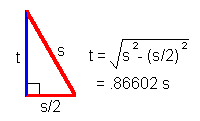Now the perpendicular distance is found by "straightening up" t. The altitude u of the upper pentagonal layer and the lower pentagonal layer is found, again, using the Pythagorean Theorem.Notice that u is equal to r... hmmm, unexpected but we'll keep going.

And finally, the distance d and angle phi may be found by the Pythagorean theorem and the law of sines.That's it, all done. That wasn't so bad, was it?

The next consideration in creating a geodesic dome is tesselation - effectively subdividing a face of a polyhedral face into subfaces. The frequency of the polyhedron indicates the number of subdivisions:

 0-frequency 0 segments per edge 02 = 0 subfaces(degeneracy: a mathematical point)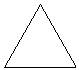1-frequency 1 segment per edge 12 = 1 subfaces(identity: the face itself)2-frequency 2 segments per edge 22 = 4 subfaces3-frequency 3 segments per edge 32 = 9 subfaces4-frequency 4 segments per edge 42 = 16 subfaces . . .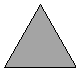n-frequency n segments per edge n2 subfaces

One of the important properties of the icosahedron is the regularity of the dimensions of its edges and faces; this is cast off when the tesselated icosahedron's vertices are projected onto the circumscribed sphere. Consider the 4-frequency face; in the following diagram color is used to indicate chords of the same length:In order of increasing length once projected, the number of components of each size are 6 red, 6 darkgreen, 3 purple, 6 orange, 6 blue, and 3 green. Out of the 30 original segments, it is clear there is significant symmetry, but just as clear is that the simplicity in manufactiuring has been reduced. This will get worse as the frequency increases.

Additionally, the subfaces now have edges of different length. In the 4-frequency face, there are five distinct shapes which can be denoted by their edges: 3 red-red-purple, 6 orange-blue-darkgreen, 3 darkgreen-darkgreen-purple, 3 blue-blue-green, and 1 green-green-green. At least many of the subfaces are bilaterally symmetric, and the rest may be flipped over if the inner and outer surfaces are the same, but it is certainly much more complex than before projection.

There is some economy of scale which has been preserved, however. Since each face is replicated 20 times on the icosahedron, each number of specialized materials can be multiplied by 20. If a dome is desired, then the multiple is lower - but still offers some economy.

Given these preliminaries, an icosahedral geodesic patch may be created by forming a two-dimensional equilateral mesh and projecting its vertices onto the circumscribed sphere. The icoshedron is part of its circumscribed sphere: each vertex lies on its surface. As the geodesic frequency increases, more and more vertices touch the sphere and it more closely the structure approximates it mathematically. However, each edge between two vertices is a straight line and each surface between three edges is a planar surface. In the limit, as n increase to infinity, the surface is the sphere.

So the remaining problem to get to a geodesic done is to calculate the lengths of the chords of the projected icosahedral geodesic patch. Only one patch needs to be calculated; it is symmetrically repeated to populate the icosahedral geodesic surface. This means we'll be duplicating the edges of the icosahedron when we duplicate the tesselated surfaces - but that's just a bother, not a problem.

To calculate the chord lengths, the mesh of vertices must be found. We'll label each vertex to facilitate it's subsequent use in chord determination.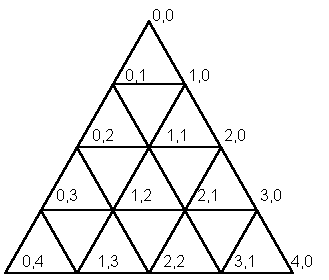Choosing one of the top faces and spinning it so the y coordinates are symetteric we can quickly determine the coordinates of each vertex in the mesh.

 Vertex x y z (0,0) 0.0 0.0 0.95105 (0,1) 0.17204 -0.125 0.81962 (1,0) 0.17204 0.125 0.81962 (0,2) 0.34409 -0.25 0.68819 (1,1) 0.34409 0.0 0.68819 (2,0) 0.34409 0.25 0.68819 (0,3) 0.51614 -0.375 0.55675 (1,2) 0.51614 -0.125 0.55675 (2,1) 0.51614 0.125 0.55675 (3,0) 0.51614 0.375 0.55675 (0,4) 0.68819 -0.5 0.42532 (1,3) 0.68819 -0.25 0.42532 (2,2) 0.68819 0.0 0.42532 (3,1) 0.68819 0.25 0.42532 (4,0) 0.68819 0.5 0.42532

Note the positions of the vertices as well as the chord lengths that we'll calculate below may all be scaled . The units in these charts correspond to scaling with an icosahedral edge length of 1. You can change that be whatever value you need it to be; the angles won't change - everything will still fit together!

Next, the vertices in the mesh must be projected from the flat equilateral triangular face of the icosahedron onto the curved surface of the circuscribed sphere. This is done by converting the mesh point to spherical coordinates, extending the radius, and converting back to Cartesian coordinates. (It's really not hard at all.)

 Vertex x y z (0,0) 0.0 0.0 0.95105 (0,1) 0.19323 -0.14039 0.92057 (1,0) 0.19323 0.14039 0.92057 (0,2) 0.40450 -0.29389 0.80901 (1,1) 0.42532 0.0 0.85065 (2,0) 0.40450 0.29389 0.80901 (0,3) 0.57971 -0.42118 0.62533 (1,2) 0.63798 -0.15450 0.68819 (2,1) 0.63798 0.15450 0.68819 (3,0) 0.57971 0.42118 0.62533 (0,4) 0.68819 -0.50000 0.42532 (1,3) 0.77295 -0.28079 0.47771 (2,2) 0.80901 0.0 0.49999 (3,1) 0.77295 0.28079 0.47771 (4,0) 0.68819 0.50000 0.42532

To find the chord lengths, the distance between adjoining pairs of vertices must be found. Distance is calculated by the 3D distance formula; a simple walk through the projected mesh looking below-left, below-right, and right will pick off all of the chords.

 Vertex Vertex chord (0,0) (0,1) 0.24079 (0,0) (1,0) 0.24079 (0,1) (0,2) 0.28397 (0,1) (1,1) 0.28011 (0,1) (1,0) 0.28079 (1,0) (1,1) 0.28011 (1,0) (2,0) 0.28397 (0,2) (0,3) 0.28397 (0,2) (1,2) 0.29755 (0,2) (1,1) 0.29755 (1,1) (1,2) 0.30901 (1,1) (2,1) 0.30901 (1,1) (2,0) 0.29755 (2,0) (2,1) 0.29755 (2,0) (3,0) 0.28397 (0,3) (0,4) 0.24079 (0,3) (1,3) 0.28079 (0,3) (1,2) 0.28011 (1,2) (1,3) 0.28011 (1,2) (2,2) 0.29755 (1,2) (2,1) 0.30901 (2,1) (2,2) 0.29755 (2,1) (3,1) 0.28011 (2,1) (3,0) 0.28011 (3,0) (3,1) 0.28079 (3,0) (4,0) 0.24079 (0,4) (1,3) 0.24079 (1,3) (2,2) 0.28397 (2,2) (3,1) 0.28397 (3,1) (4,0) 0.24079

Or, the chords may be collected in a bill of materials.

 Chords count chord (0,0)-(0,1) (0,0)-(1,0) (0,3)-(0,4) (3,0)-(4,0) (0,4)-(1,3) (3,1)-(4,0) 6 0.24079 (0,1)-(1,1) (1,0)-(1,1) (0,3)-(1,2) (1,2)-(1,3) (2,1)-(3,1) (2,1)-(3,0) 6 0.28011 (0,1)-(1,0) (0,3)-(1,3) (3,0)-(3,1) 3 0.28079 (0,1)-(0,2) (1,0)-(2,0) (0,2)-(0,3) (2,0)-(3,0) (1,3)-(2,2) (2,2)-(3,1) 6 0.28397 (0,2)-(1,2) (0,2)-(1,1) (1,1)-(2,0) (2,0)-(2,1) (1,2)-(2,2) (2,1)-(2,2) 6 0.29755 (1,1)-(1,2) (1,1)-(2,1) (1,2)-(2,1) 3 0.30901

Here are the first five tesselations for an icosahedral patch:

and here's a coffeescript app to generate the chords for an arbitrary frequency and edge length.